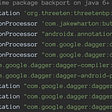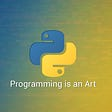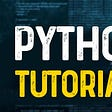# Python Algorithm: Pt. 12: GREEDY >:D

1. Class time!
`def greedy(course):length = len(course)course_list=[]course_list.append(course)course_end_time = course_listfor i in range(1, length):if course[i] >= course_end_time:course_list.append(course[i])course_end_time = course[i]return course_listcourse = {'Biology':(12, 13),'English':(9, 11),'Math':(8, 10),'IT':(10, 12),'Physics':(11,13),}cs = sorted(course.items(), key=lambda item:item)print('IDK')print('Class', '    Starting time', 'FINISH CLASS!')for i in range(len(cs)):print("{0}{1:7d}:00{2:8d}:00".format(cs[i],cs[i],cs[i]))s=greedy(cs)print("IDK")print("Class", "   Starting time", "FINISH CLASS!")for i in range(len(s)):print("{0}{1:7d}:00{2:8d}:00".format(s[i],s[i],s[i]))`
`def greedy(things):length = len(things)things_list = []things_list.append(things[length-1])weights = things[length-1]for i in range(length-1, -1, -1):if things[i] + weights <= max_weight:things_list.append(things[i])weights += things[i]return things_listthings = {'iWatch':(15000, 0,1),'Asus':(35000, 0,7),'iPhone':(38000, 0,3),'Acer':(40000, 0,8),'Go Pro':(12000, 0,1),}max_weight = 1th = sorted(things.items(), key=lambda item:item)print("IDK")print('Product', '                Price', 'Weight')for i in range(len(th)):print("{0:8s}{1:10d}{2:10.2f}".format(th[i],th[i],th[i]))t = greedy(th)print("IDK")print("Product", "                            Price", "Weight")for i in range(len(t)):print("{0:8s}{1:10d}{2:10.2f}".format(t[i],t[i],t[i]))`

--

--

--

## More from Che Kai LIANG

Love podcasts or audiobooks? Learn on the go with our new app.

## SUBSTR() Character Function — Oracle SQL Example## Local To-Dos for Developers## Role of Documentation and Software Architecture in Cubix## Easy mysqldump backups by example.## Graceful shutdown in go## Scoping Activities Subcomponents with dagger-android## Ten features you can build for your server-side testing tool## Q#63 Calculating a moving average — using python## Single underscore and Double underscore in Python.## Find The Needles: Python Version## Introduction to Python Programming Part 3— Containers